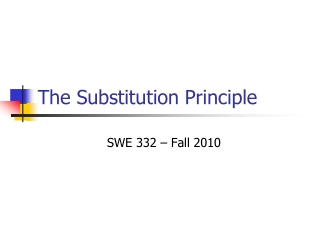DownloadDownload PresentationThe Substitution Principle

# The Substitution Principle

Télécharger la présentation## The Substitution Principle

- - - - - - - - - - - - - - - - - - - - - - - - - - - E N D - - - - - - - - - - - - - - - - - - - - - - - - - - -
##### Presentation Transcript

1. The Substitution Principle SWE 332 – Fall 2010

2. Liskov Substitution Principle In any client code, if subtype object is substituted for supertype object, the client’s expectations are still met • Simple example: Object o = getNewObject(); // client call • Case 1: public Object getNewObject() {...} • Case 2: public String getNewObject() {...}

3. Dynamic Dispatching Object[] x = new Object; X = new String(“abc”); X = new Integer(1); for(int i=0; i<x.length;i++) System.out.println(x[i].toString()); • Compiler does not complain • Apparent type is fine! • Which toString method is called? • Object.toString() • String.toString() • Integer.toString() • At run time, “best fit” code is called.

4. Two valid reasons to subtype • Multiple implementations • Ideally, client unaware of multiple implementations • Example: • Set interface • HashSet, TreeSet implementations • Extended Behavior • Usual reason for subtyping • Note what is not a reason: • Making implementer’s life easier

5. Vehicle Car Bike Extended behavior • Extended Behavior • Specialize the behavior of supertype • Classic ‘IS A’ relationship • Additional state (abstract or representation) • Warning: Harder than it looks! CAR Vehicle Constraint View: for contracts Object View: for rep

6. Analyzing subtype METHODS • Subtypes behavior must support supertype behavior • Liskov Substitution Principle • Three rules for subtype methods: • Signature Rule • Methods Rule • Properties Rule

7. Signature Rule • Guaranteed by Compiler • Subtypes must have all methods of supertype • Sounds obvious, but programmers often try to get around this • Signatures of methods must be compatible with supertype signature • Typically, return types are the same • Covariance: Subclass may return a subtype • Exceptions: • Signature Rule allows fewer exceptions • But Methods Rule may be in conflict • Methods Rule always has priority

8. More concretely… public class (alt. interface) Super{ /** * m() is defined … (pre) * m() does the following things (post) * @throws … (post) */ public T1 m (T2: t2, T3: t3) throws E1, E2… } public class Sub extend (alt. implements) Super{ /** ??? */ public T1 m(T2: t2, T3: t3) throws E1, E2… }

9. Methods Rule • When object belongs to subtype, subtype method is called • Supertype specifications are necessarily inherited • For analysis, consult pre/post • See prior slide

10. Methods Rule • Must maintain the contract! • Precondition rule: What can a subclass do with preconditions in supertype spec? • Post condition rule: What can a subclass do with postconditions in supertype spec?

11. Precondition rule • Subtype is allowed to weaken the precondition • Formally: • If pre_super, then pre_sub • Super //pre x > 5 • Case 1: Sub //pre x > 6 • Case 2: Sub // pre x > 4 • x>5  x>4? Which is weaker? • x>5  x>6? • Not checked by compiler

12. Post condition rule • Subtype is allowed to strengthen the post condition in a consistent way • Formally: • If pre_super, and sub_post, then super_post • Super: // post: returns y < 5 • Sub: //post: returns y < 4 • Sub: //post: returns y < 6 • Which one is a stronger condition?

13. Same Diagram as Method Verification Supertype State (Pre-Super) Supertype State (Post-Super) SuperType Method Contract ? AF() AF() Subtype State (Post-Sub) Subtype State (Pre-Sub) Subtype Method Contract

14. Super public void addZero() //pre: this is not empty //post: add zero to this public void addZero() throws ISE //pre: this is not empty //post: add zero to this Sub public void addZero() //post: add zero to this public void addZero() throws ISE //post: if this is empty, throw ISE else add zero to this Examples Satisfies Signature and Method rules Satisfies Signature and Method rules

15. Super public void addZero() //pre: this is not empty //post: add zero to this public void addZero() throws ISE //post: if this is empty, throws ISE // else add zero to this Sub public void addZero() throws ISE //post: add zero to this public void addZero() //post: add zero to this More examples Does not satisfy Signature rule Does not satisfy Postcondition part of methods rule

16. Client code : Liskov Substitution Principle private void foo { … try{ o.addZero(); } catch (ISE e){ //do something: Client expects to get here! } }

17. Methods rule vs. Properties rule • Methods rule is for single method invocation • Properties rule about general object behavior • Invariants: • Example: Sets do not contain duplicates • Evolution properties: • Example: Monotone sets only grow • No remove() method allowed • Properties must be explicit (i.e. in the JavaDoc)

18. More About Properties Rule Collection <String> c = ...; c.add (“cat”); c.add (“cat”); c.remove(“cat”); // consider the following observer call: // What is behavior if c is a Set? // What is behavior if c is a Bag? if (c.contains(“cat”) { ... } // Such “algebraic” relations are extremely useful for testing